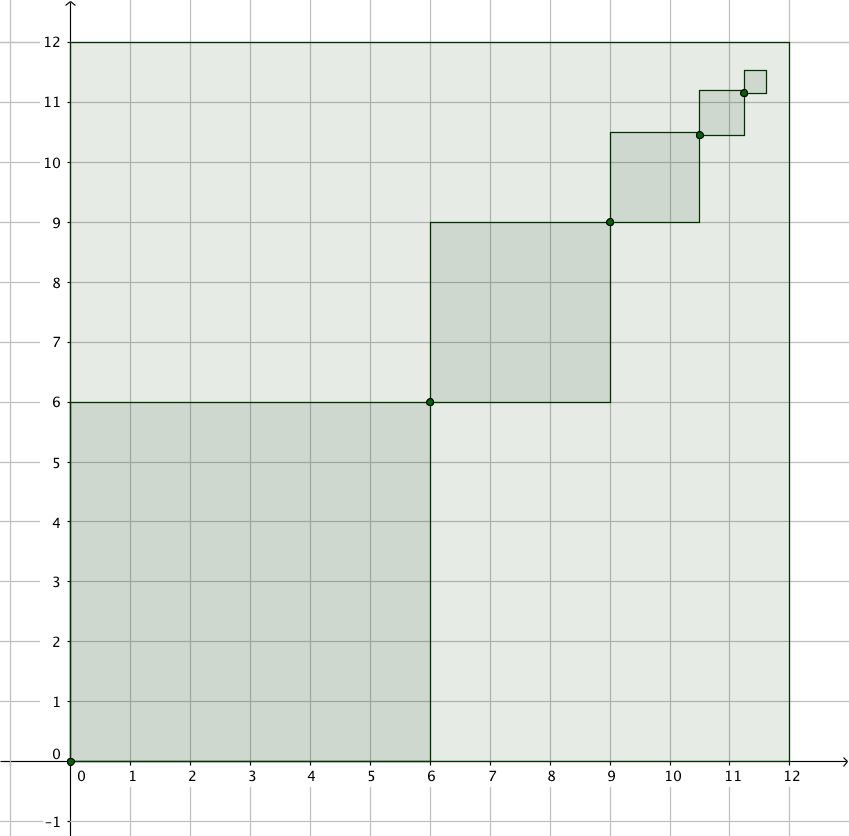# Introduction

In the following image, a square of length 12 units has been drawn. A sequence of squares has been drawn within the square.Use the applet below to recreate the sequence of squares, and to continue drawing squares towards the top right hand corner.

To use the square tool, first plot a point for the bottom left vertex of the square. Then select the square tool. Select the point, then enter a side length.

# Investigate

The area of the larger square is 144 cm.

What is the area of the first square of the sequence?

What portion of the square is the first square of the sequence?

What is the total area of the first 5 squares within the large square?

What portion of the large square do the first 5 squares cover?

What about the first 10 squares? etc

Now the area of the smaller squares added together cannot exceed 144 units. Why not? We could say thatis an upper bound for this value. What other number could serve as an upper bound for this sequence, that is smaller than? Why?

What number could serve as a lower bound for the sum of the sequence of the areas of the squares?